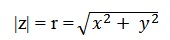Study Materials: CBSE Notes

Our CBSE Notes for Polar representation of a complex number Class 11 maths 5. Complex Number and Quadratic Equations - CBSE Notes - Toppers Study is the best material for English Medium students cbse board and other state boards students.

Notes ⇒ Class 11th ⇒ Mathematics ⇒ 5. Complex Number and Quadratic Equations

# Polar representation of a complex number Class 11 maths 5. Complex Number and Quadratic Equations - CBSE Notes - Toppers Study

Topper Study classes prepares CBSE Notes on practical base problems and comes out with the best result that helps the students and teachers as well as tutors and so many ecademic coaching classes that they need in practical life. Our CBSE Notes for Polar representation of a complex number Class 11 maths 5. Complex Number and Quadratic Equations - CBSE Notes - Toppers Study is the best material for English Medium students cbse board and other state boards students.

## Polar representation of a complex number Class 11 maths 5. Complex Number and Quadratic Equations - CBSE Notes - Toppers Study

CBSE board students who preparing for class 11 ncert solutions maths and Mathematics solved exercise chapter 5. Complex Number and Quadratic Equations available and this helps in upcoming exams 2023-2024.

### You can Find Mathematics solution Class 11 Chapter 5. Complex Number and Quadratic Equations

• All Chapter review quick revision notes for chapter 5. Complex Number and Quadratic Equations Class 11
• NCERT Solutions And Textual questions Answers Class 11 Mathematics
• Extra NCERT Book questions Answers Class 11 Mathematics
• Importatnt key points with additional Assignment and questions bank solved.

NCERT Solutions do not only help you to cover your syllabus but also will give to textual support in exams 2023-2024 to complete exercise maths class 11 chapter 5 in english medium. So revise and practice these all cbse study materials like class 11 maths chapter in english ncert book. Also ensure to repractice all syllabus within time or before board exams for ncert class 11 maths ex in english.

See all solutions for class 11 maths chapter 5 exercise 5 in english medium solved questions with answers.

### Polar representation of a complex number class 11 Mathematics Chapter 5. Complex Number and Quadratic Equations

• Polar Representation Of A Complex Number Class 11 Maths 5. Complex Number And Quadratic Equations - CBSE Notes - Toppers Study
• Class 11 Ncert Solutions
• Solution Chapter 5. Complex Number And Quadratic Equations Class 11
• Solutions Class 11
• Chapter 5. Complex Number And Quadratic Equations Polar Representation Of A Complex Number Class 11

## Polar representation of a complex number Class 11 maths 5. Complex Number and Quadratic Equations - CBSE Notes - Toppers Study

Polar representation of a complex number:

Argand plane: The plane having a complex number assigned to each of its point is called the complex plane or the Argand plane.

Polar form: Polar form of a complex number is another way to represent a complex number on argand plane.

if z = x + iy is any complex number then

In polar representation a complex number z is represented by two parameters r and θ. Where parameter r is the modulus of a complex number and θ is the angle with the positive direction of x - axis.using pythagoros theorem

Here Z of modulus = r and θ is called the argument (or amplitude) of z which is denoted by arg z.

Principle arguments of z : The value of θ such that – π < θ ≤ π, called principal argument of z and is denoted by arg z.

The point (x,y) represent a normal cartesian coordinate. But in polar form this point is reoresented by a special coordinate system which is called polar coordinate having (rθ).

This coordinate (rθ) represents the each location of a point of a complex number.

So, We have there is a relation between polar cordinates and cartesian coordinates.We know;

z = Re(z) + Img(z)

Then x-axis represent Re(z) and y-axis represent Img(z).

We consider Origin (0, 0) as pole.

For any complex number z = x + iy is represented as r (cosθ + i sinθ) as any point on complex plane. This is called polar representation of a complex number. Where θ is angle between r and x-axis.

Important Study materials for classes 06, 07, 08,09,10, 11 and 12. Like CBSE Notes, Notes for Science, Notes for maths, Notes for Social Science, Notes for Accountancy, Notes for Economics, Notes for political Science, Noes for History, Notes For Bussiness Study, Physical Educations, Sample Papers, Test Papers, Mock Test Papers, Support Materials and Books.Mathematics Class - 11th

NCERT Maths book for CBSE Students.

books

## Study Materials List:

##### Notes ⇒ Class 11th ⇒ Mathematics
1. Sets
2. Relations and Functions
3. Trigonometric Functions
4. Principle of Mathematical Induction
5. Complex Number and Quadratic Equations
6. Linear Inequalities
7. Permutations and Combinations
8. Binomial Theorem
9. Sequences and Series
10. Straight Lines
11. Conic Sections
12. Introduction to Three Dimensional Geometry
13. Limits and Derivatives
14. Mathematical Reasoning
15. Statistics
16. Probability

New Books